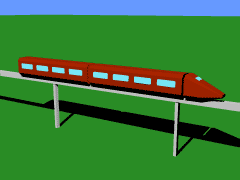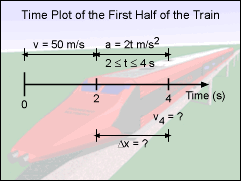Ch 1. Particle General Motion Multimedia Engineering Dynamics Position,Vel & Accel. Accel. varyw/ Time Accel. Constant Rect. Coordinates Norm/Tang. Coordinates Polar Coordinates RelativeMotion
 Chapter - Particle - 1. General Motion 2. Force & Accel. 3. Energy 4. Momentum - Rigid Body - 5. General Motion 6. Force & Accel. 7. Energy 8. Momentum 9. 3-D Motion 10. Vibrations Appendix Basic Math Units Basic Equations Sections Search eBooks Dynamics Fluids Math Mechanics Statics Thermodynamics Author(s): Kurt Gramoll ©Kurt GramollDYNAMICS - CASE STUDY IntroductionTrain Motion A high-speed train is traveling at 50 m/s when the first half of the train, which includes the engine, breaks away from the second half. Due to the loss in weight and drag, the first half of the train accelerates for two seconds before the engineer regains control. What is known: The train is moving along a linear path. The train had been traveling at 50 m/s for 2 seconds when the break occurs. The acceleration of the first half of the train after the break at t = 2 sec is a(t) = 2t m/s2         2 ≤ t ≤ 4 secondsTrain conditions over time Question Knowing the acceleration of the train as a function of time after the break, what is the velocity of the first half of the train at t = 4 seconds, and how far does it travel from t = 2 to t = 4 seconds? Approach Begin begin with an equation for the acceleration and integrate, rather than begin with an equation for the position and differentiate as was done in the Position, Velocity, Acceleration section. Integrate the basic acceleration equation (a = d2x/dt2) to find expressions for the velocity and position of the train.

Practice Homework and Test problems now available in the 'Eng Dynamics' mobile app
Includes over 400 problems with complete detailed solutions.
Available now at the Google Play Store and Apple App Store.